[BACK]
 Computers, Materials & ContinuaDOI:10.32604/cmc.2021.018523Article

Adaptive Error Curve Learning Ensemble Model for Improving Energy Consumption Forecasting

Department of Computer Engineering, Jeju National University, Jeju-si, Korea
*Corresponding Author: Yung-Cheol Byun. Email: ycb@jejunu.ac.kr
Received: 10 March 2021; Accepted: 11 April 2021

Abstract: Despite the advancement within the last decades in the field of smart grids, energy consumption forecasting utilizing the metrological features is still challenging. This paper proposes a genetic algorithm-based adaptive error curve learning ensemble (GA-ECLE) model. The proposed technique copes with the stochastic variations of improving energy consumption forecasting using a machine learning-based ensembled approach. A modified ensemble model based on a utilizing error of model as a feature is used to improve the forecast accuracy. This approach combines three models, namely CatBoost (CB), Gradient Boost (GB), and Multilayer Perceptron (MLP). The ensembled CB-GB-MLP model’s inner mechanism consists of generating a meta-data from Gradient Boosting and CatBoost models to compute the final predictions using the Multilayer Perceptron network. A genetic algorithm is used to obtain the optimal features to be used for the model. To prove the proposed model’s effectiveness, we have used a four-phase technique using Jeju island’s real energy consumption data. In the first phase, we have obtained the results by applying the CB-GB-MLP model. In the second phase, we have utilized a GA-ensembled model with optimal features. The third phase is for the comparison of the energy forecasting result with the proposed ECL-based model. The fourth stage is the final stage, where we have applied the GA-ECLE model. We obtained a mean absolute error of 3.05, and a root mean square error of 5.05. Extensive experimental results are provided, demonstrating the superiority of the proposed GA-ECLE model over traditional ensemble models.

Keywords: Energy consumption; meteorological features; error curve learning; ensemble model; energy forecasting; gradient boost; catboost; multilayer perceptron; genetic algorithm

1  Introduction

Predicting energy consumption remains a problematic and mathematically demanding task for energy grid operators. Current prediction methods are typically based on a statistical analysis of the load temperature observed in various channels and generating a warning if a critical threshold is reached. However, the latest computer science advances have shown that machine learning can be successfully applied in many scientific research fields, especially those that manipulate large data sets . Many researchers have proposed using the residual compensation method to improve the accuracy. Such as Su et al.  presented an improved collaborative research framework for predicting solar power generation. An enhanced collection model is being proposed to improve accurate predictions based on a new waste adaptation solution evolutionary optimization technique. They applied this solution to the solar projection using an asymmetric estimator. They conducted extensive case studies on open data sets using multiple solar domains to demonstrate Adaptive Residual Compensation integration technology’s benefits. In this article by Christian et al. , the authors have proposed a new method of predicting thunderstorms using machine learning model error as a feature. Their work’s basic idea is to use the two-dimensional optical flow algorithm’s errors applied to meteorological satellite images as a function of machine learning models. They interpret that an error in the speed of light is a sign of convection and can lead to thunder and storms. They wrap using various manual steps to consider the proximity to space. They practice various tree classification models and neural networks to predict lightning over the next few hours based on these characteristics. They compared the effects of different properties on the classification results to the predictive power of other models. Another work of using machine learning model’s error is done by Zhang et al. . The authors proposed a way to train a single hidden layer feed-forward neural network using an extreme learning machine. The proposed method presented found an effective solution to the regression problem due to the limited modeling ability, the problem’s nonlinear nature, and the regression problem’s possible nature. Extreme learning machine-based prediction error is unavoidable due to its limited modeling capability. This article proposes new Extreme learning machines for regression problems, such as the Extreme learning machine Remnant Compensation, which uses a Multilayered framework that uses baselines to compensate for inputs and outputs other levels of correction. Post-Layer Levels The proposed residual compensation-based-extreme learning machine may also be a general framework for migration issues. However, they did not combine the proposed approach with deep learning schemes.

We have proposed a modified ensemble model to improve the forecast accuracy based on utilizing the model’s error as a feature. This approach combines three models, namely CatBoost (CB), Gradient Boost (GB), and Multilayer Perceptron (MLP). The ensembled CB-GB-MLP model’s inner mechanism consists of generating a meta-data from Gradient Boosting and CatBoost models to compute the final predictions using the Multilayer Perceptron network. A genetic algorithm is used to obtain the optimal features to be used for the model. To prove the proposed model’s effectiveness, we have used a four-phase technique using South Korea’s Jeju province’s actual energy consumption data. Jeju Island is located on the southernmost side of the Korean peninsula. The solar altitude remains high throughout the year, and in summer, it enters the zone of influence of tropical air masses. It is situated in the Northwest Pacific Ocean, which is the Pacific Ocean’s widest edge and is far from the Asian continent and is affected by the humid ocean . The foremost contributions of this article are to

•   combine three models of machine learning, namely Catboost, Gradient Boost, and Multilayer Perceptron,

•   utilizing a genetic algorithm for the feature selection,

•   using error of model as a feature to improve the forecast accuracy.

The remainder of the article is arranged as follows. Section 2 introduces preliminaries about machine learning techniques used in this publication. Section 3 presents the four-stage proposed methodology. Section 4 introduces the data collection, data analysis process, pre-processing, and training. Section 5 presents the performance results of the proposed model evaluated using Jeju energy consumption data. It also analyzes the results with the existing models. Lastly, we conclude this article in the last conclusion section.

2  Preliminaries

Artificial neural networks and machine learning provide better results than traditional statistical prediction models in various fields. Li  proposed a short-term forecasting model that included extensive data mining and several continuous forecasting steps. To reduce the hassle with noise in the short term, noise reduction methods are used based on analysis and reconstruction. The phase reconstruction method is used to determine the dynamics of testing, training, and neuron configuration of the artificial neural network (ANN). It also improves the ANN parameter by applying a standard grasshopper optimization algorithm. The simulation results show that the proposed model can predict the load in the short term using various measurement statistics. However, other factors or parameters can be considered in the forecasting model to optimize the short-term load prediction. The focus should be on developing data processing technology that can manage short, erratic, and unstable data so users can manage the negative effects of noise. We have proposed to ensemble three machine learning models followed by the genetic algorithm optimization technique. This section introduces these three ML models according to their distinguished architecture and uses in literature, namely, Gradient Boosting model, CatBoost Model, and Multilayer perceptron. Moreover, the Genetic algorithm and Ensemble model approaches are also discussed.

Gradient Boosting model is a robust machine learning algorithm developed for various data such as computer vision , chemical , and biological fields , and energy . Tree-based ensemble methods are gaining popularity in the field of prediction. In general, combining a simple low return regression tree gives high accuracy of forecasting. Unlike other machine learning techniques, Tree-based ensemble methods are considered black boxes. Tree-based grouping techniques provide interpretable results, have little data processing, and handle many different types of predictions. These features make tree-based grouping techniques an ideal choice for solving travel time prediction problems. However, the application of the tree-based clustering algorithm in traffic prediction is limited. In the article, Zhang et al.  use the Gradient Boosted Regression Tree method to analyze and model highway driving time to improve prediction accuracy and model interpretation. The gradient boosting method could strategically combine different trees with improving prediction accuracy by correcting errors generated by the previous base model. They discussed the impact of other parameters on model performance and variable input/output correlations using travel time data provided by INRIX on both sections of the Maryland Highway. They compared the proposed method with other hybrid models. The results show that gradient boosting has better performance in predicting road transit times.

In the study, Touzani et al. , a modeling method based on motor power consumption using gradient boosting algorithm, is proposed. A recent testing program has been used in an extensive data set of 410 commercial buildings to assess this method’s effectiveness. The model training cycle evaluates the sample performance of different predictive methods. The results show that the use of a gradient boosting compared to other machine learning models improves R-square and root mean square error estimates by more than 80%.

2.2 Catboost Model

CatBoost is a gradient boosting algorithm-based open-source machine learning library. It can successfully adjust the categorical features and use optimization instead of adjusting time during training . Another advantage of this algorithm is that it reduces the load and uses a new system to calculate the leaves’ value in determining the shape of the tree . It is mainly used to solve logical problems and to solve functions efficiently. High-quality samples can be obtained without parameter adjustment, and better results can be obtained by using a default parameter, thereby reducing the conversion time. It also supports categorical parts without pre-fixing non-numerical properties. In work by Diao et al. , catboost is used for short-term weather forecasting using the datasets provided by Beijing Meteorological Administration. The corresponding heat map, the removal of the recursive features, and the tree pattern are included in selecting the features. Then they recommended the wavelet denoising of data and conducted it before setting up a learning program. The test results show that compared to most in-depth studies or machine learning methods, the catboost mode can shorten the connection time and improve the system.

2.3 Multi-Layer Perceptron

The multi-layer perceptron (MLP) consists of simple systems connected to neurons or nodes. The nodes involved in the measurement and output signals result from the amount of material available for that node, which is configured by the function or activation of a simple nonlinear switch . This is a feature of a straightforward nonlinear transition, allowing nonlinear embedding functions in a multi-layer perceptron. The output is measured by connection weight and then sent to the node in the next network layer as an input. The multi-layer perceptron is considered a feed-forward neural network. In the study by Saha et al. , a multi-layer perceptron model was used to predict the location of potential deforestation. Group-level success rates and levels are compared to MLP. The described unit has been trained on 70% of deforestation; the remaining 30% have been used as test data. Distance to settlement, population growth, and distance to roads were the most important factors. After assembling the MLP neural network calibration with the hybrid classifier, the accuracy is increased. The described method can be used to predict deforestation in other regions with similar climatic conditions.

The MLP algorithm is trained to calculate and learn the weights, synapses, and neurons of each layer . MLP can be calculated using Eq. (1).

yk=α(i=1n(ωi,kyi+ωk))(1)

where the sigmoid function is α, ω is the weight, and k denotes the multi-layer perceptron model’s output value, whereas yk is the output of the MLP. yi is given as the input for the first layer to obtain the kth output. i indicates the number of the hidden layer from one to n. wi;k is the connection between the ith hidden layer neuron and the kth output layer neuron.

2.4 Genetic Algorithm

Genetic algorithm (GA) was inspired by Darwin’s theory of evolution, in which the survival of organisms and more appropriate genes were simulated. GA is a population-based algorithm. All solutions correspond to the chromosomes, and each parameter represents a gene . GA uses the (objective) fitness function to assess the fitness of everyone in the population. To improve the poor solution, it is randomly selected using the optimal solution selection mechanism. The GA algorithm starts with a random set. Populations can be generated from Gaussian random distributions to increase diversity. Inspired by this simple natural selection idea, we use the GA-algorithm roulette wheel to select them to create a new generation that matches the fitness value assigned to an individual with probability. After choosing an individual with a limiting factor, this is used to create a new generation. The last evolutionary factor in which one or more genes are altered after making an eye solution. The mutation rate is set low because the high GA mutation rate translates GA into a primitive random search term . The mutation factor maintains the diversity of the population by introducing different levels of randomness.

2.5 Ensemble Model

Ensemble refers to the integration of prediction models to perform a single prediction. Ensemble models can combine different weak predictive models to create more robust predictive models . The Blending Ensemble model uses not only the predicted value but also the original feature. Fig. 1 shows the Graphical representation of the ensemble machine learning approach. Where the dataset is training in different models, and their initial predictions are ensembled using ensembler. In the article by Massaoudi et al. , the authors propose a framework by using Light Gradient Boosting Machine (LGBM), eXtreme gradient Boosting machine (XGB), and Multilayer Perceptron (MLP) for calculating effective short-term forecasts. The proposed method uses the heap generalization method to identify potential changes in housing demand. The proposed method is the combination of three models LGBM, XGB, and MLP. The Stacked XGB-LGBM-MLP model’s inner mechanism consists of producing metadata from XGB and LGBM models to compute the final predictions using the MLP network. The performance of the proposed XGB-LGBM-MLP stack model is tested using two data sets from different sites. The proposed method succeeded in reducing errors; however, the performance of the proposed method of stacking would decrease up to 48 h slower.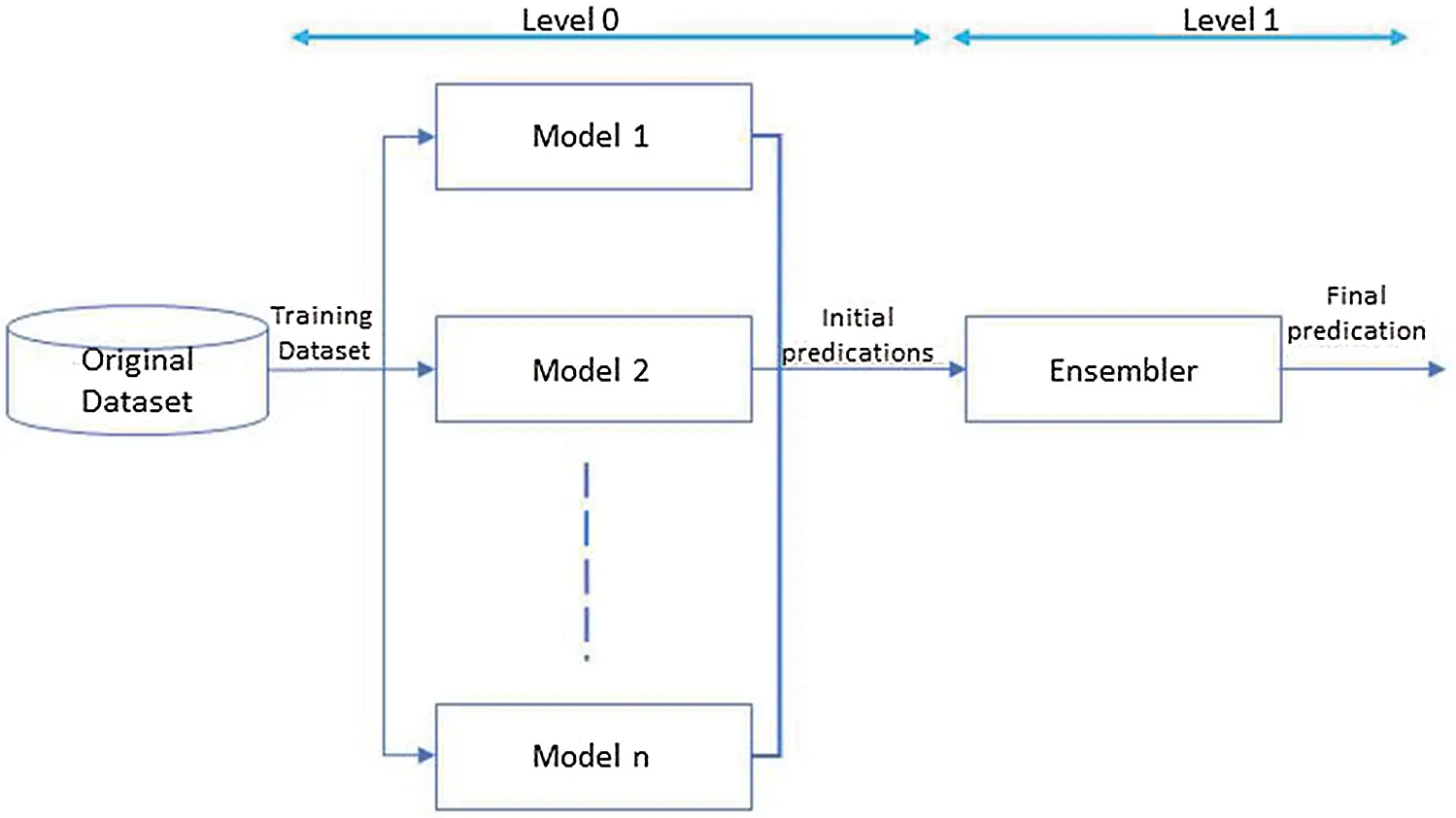Figure 1: Graphical representation of the ensemble machine learning approach

3  Proposed Methodology

We have designed a four-phase strategy better to understand the impact of the error curve learning technique. Fig. 2 shows the abstract of these four phases. In the first phase, we have obtained the results by applying the CB-GB-MLP model. This approach combines three models, namely CatBoost (CB), Gradient Boost (GB), and Multilayer Perceptron (MLP). The ensembled CB-GB-MLP model’s inner mechanism consists of generating a meta-data from Gradient Boosting and CatBoost models to compute the final predictions using the Multilayer Perceptron network. In this phase, 70% of the original dataset is used for training purposes and 30% for testing. In the second phase, we have utilized a GA-ensembled model with optimal features. The Input feature for energy consumption forecasting consists of weather, time, and holidays.Figure 2: Design of the four-phase strategy

A genetic algorithm is used to obtain optimal features. The CB-GB-MLP model is then used for the forecasting using the same data division scheme as used in phase 1. By applying the GA-ensembled model, we obtained better performance results. By using Eq. (2), the error curve is obtained at this stage. Where yt is the actual value of energy consumption and y^tis the predicted value.

ECt=yt+y^tyt×100(2)

The third phase is for the comparison of the energy forecasting result with the proposed ECL-based model. In this stage, we have used the testing dataset of phase 2 as the complete dataset. Then we split this data into 70% and 30% for training and testing, respectively.

The fourth stage is the final stage, where we have applied the genetic algorithm-based error curve learning ensembled (GA-ECLE) model. For this phase, we have again used the testing dataset of phase 2 as the complete dataset. Then we split this data again into 70% and 30% for training and testing, respectively. However, this time we have used the error data obtained at phase 2 as an input feature along with weather, time, and holiday features. By utilizing the GA-ECLE model, we obtained comparatively good results. Fig. 3 explains the flow diagram of the proposed GA-ECLE model. It starts with acquiring the input data from different sources, then doing some feature engineering such as obtaining date features and then pre-processing it. Preprocessing involves filling the missing values and converting the textual data into numeric data for better training. The pre-processed data is then split into training and testing datasets. The genetic algorithm is used to obtain optimal features; then, these optimal features are passed to an ensembled model where test data is used to generate the error curve. The ensemble model is trained again using the error curve and then obtaining the ensembled model’s optimal predictions.

y(t+1)=f(y(t),,y(t-m+1):F(3)

F=WFts,DFt,HFt,EFt(4)

Time series data is used to evaluate the performance of the proposed model. Eq. (3) is used to estimate the predicted value of the next timestamp. The data we have used is hourly based, so here t represents one Hour of time. y(t + 1) is the forecasted value at a given time, y(t) represents the hourly load consumption at time t, and F represent the Features used to estimate the future load value. As expressed in Eq. (4) there are four different classes of features used. Where WFts,DFt,HFt, and EFt represents the weather, date, holiday, and error features, respectively.

There are four different weather stations in Jeju island named as Jeju-Si, Gosan, Sungsan, and Seogwipo. Set of weather features from each weather station WFts, is represented in Eq. (5). Meteorological features consist of Average temperature TAt, the temperature of dew point TDt, humidity HMt, wind speed WSt, wind direction degree WDt, atmospheric pressure on the ground PAt discomfort index DIt, sensible temperature STt, and solar irradiation quantity SIt. Each weather station is assigned a different weight factor according to its importance as described in Eq. (6). Where the value of a is equal to 0:5, b and g is equal to 0:1 and d is 0:3. WFtj,β.WFtg,γ.WFtss,andδWFtsp represent the set of weather features, according to the weather stations of Jeju-Si, Gosan, Sungsan and Seogwipo respectively.

WFts={TAt,TDt,HMt,WSt,WDt,PAt,DIt,STt,SIt}(5)

WFts=α.WFtj,β.WFtg,γ.WFtss,δWFtsp(6)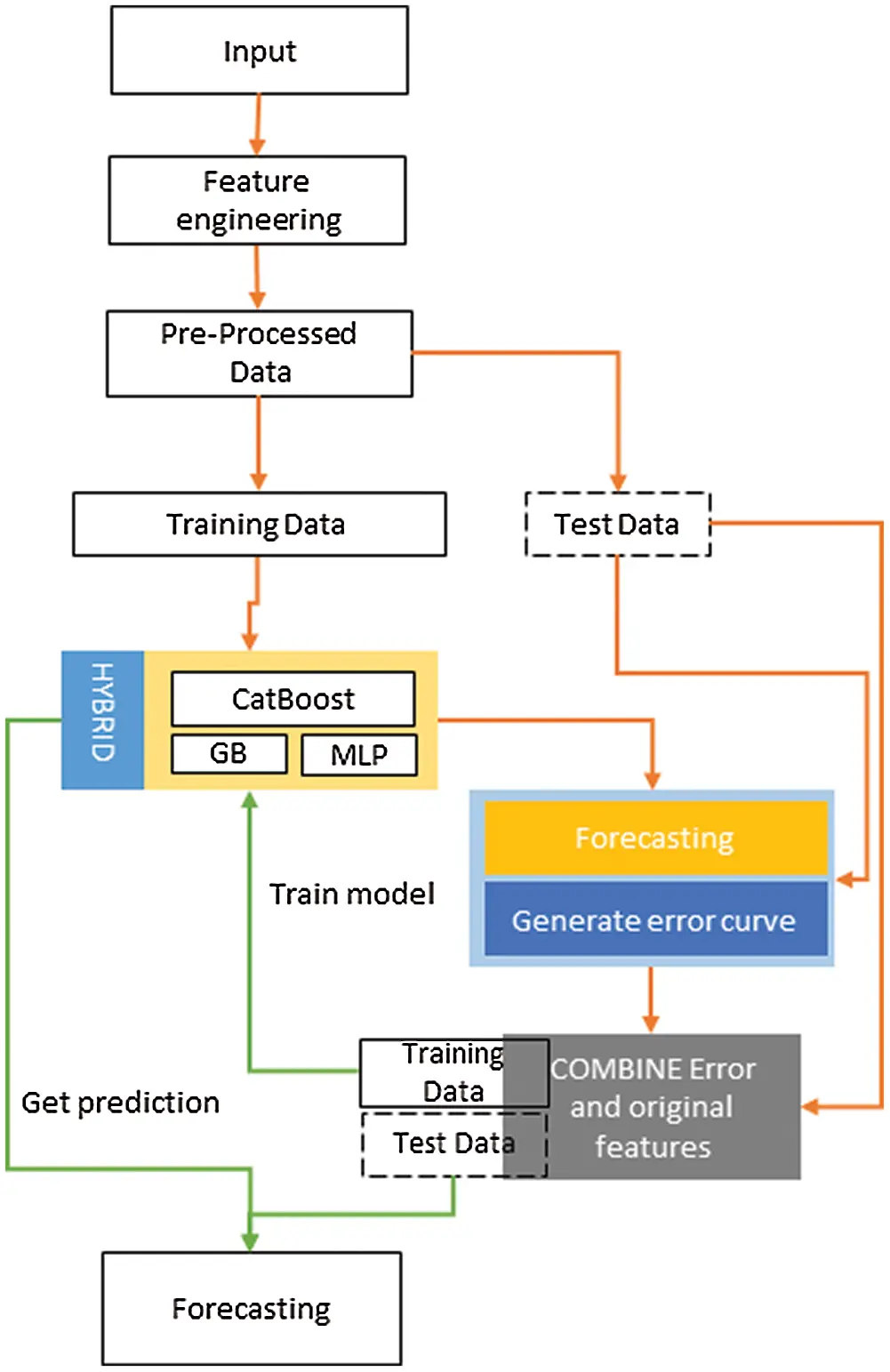Figure 3: Flow diagram of the proposed GA-ECLE framework

Date features DFt can be extracted from the time-series data, which helps understand the relation between the target value with respect to the time . The set of date features as described in Eq. (7) consist of Hour Ht,, month Mt,, year Yt,, Quarter Qt, and day of week DoWt,. Holidays also greatly impact energy consumption; we have collected the holidays and make a set of holiday features HFtas expressed in Eq. (8).

DFt={Ht,Mt,Yt,Qt,DoWt,}(7)

HFt={HDt,SDCt,SDNt,}(8)

It contains holiday code HDt,, special day code SDCt,, special day name SDNt,. Holiday code consists of holidays such as solar holiday, lunar holiday, election day, holidays interspersed with workdays, alternative holidays, change in demand, and special days. Special days consist of several holidays, including New Year’s Day, Korean army day, Korean New Year’s Day, Christmas, workers’ day, children’s day, constitution day, and liberation day.

EFt={ECt,AECt,}(9)

AECt=|yt+y^tyt|×100(10)

We have proposed to use the error of model EFt as a feature. It contains two features as explained in Eq. (9). ECt is calculated using Eq. (2) and absolute error curve AECt using Eq. (10).

Lttotal=Ltff+Ltbtm+Ltpv+Ltwp(11)

The target feature is the total hourly based load consumption Lttotal. Jeju island fulfills its energy need from different sources. These sources include renewable and non-renewable energy sources such as wind energy and solar energy. We have combined all the load consumption energy sources using Eq. (11). Where Ltff shows the energy load using fossil fuel-based energy sources, Ltbtm is for behind the meter sources. Ltpv  represents load from photovoltaic sources and load consumption from wind energy sources are represented as Ltwp.

ythybrid=Cw.ytcb+Cw.ytgb+Cw.ytmlp(12)

Cw=min(t=1M(yt-y^t)),0Cw1(13)

The proposed hybrid model consists of three sub-models, Catboost, gradient boost, and multi-layer perceptron. The output of p roposed hybrid model ythybrid is calculated using Eq. (12). Where ytcb; ytgb; ytmlprepresents the output of Catboost, gradient boost and multi-layer perceptron models, respectively. Cw represent the weight coefficient of each model and it is calculated using Eq. (13). In this Eq. time t consist of M values,yt is the actual value and ŷt is the predicted output.

4  Forecasting Using GA-ECLE Model

This section explains the complete data acquisition, data analysis, feature engineering, and proposed model training. Fig. 4 shows the block diagram of the proposed forecasting framework in the context of the Jeju island’s weather and energy consumption patterns. We have used the actual energy consumption data of Jeju island South Korea. This island has four weather stations named Jeju-si, gosan, sungsan, and seogwipo. Input variables for this model are of four different types. First is the meteorological features from four different weather stations. The second is holidays, the third is time features, and the Fourth is energy sources, which comprise of fossil fuel-based energy sources (FF), photovoltaic (PV), behind-the-meter (BTM), and wind power (WP) energy sources.Figure 4: Block diagram of the proposed forecasting framework

Prepossessing this data involves different functions such as data cleaning, one-hot encoding, imputation, and feature construction. We have also assigned different weights to the weather features according to the impact of each weather station. This pre-processed data is used as input for the genetic algorithm, which helps obtain optimal features according to their importance in prediction. The initial number of features was 64, and it was reduced to 32 after applying the genetic algorithm. We provided error and absolute error as features along with other holidays, meteorological, and date features. These features served as input to the ECLE model. This enabled model consists of three models, namely CatBoost (CB), Gradient Boost (GB), and Multilayer Perceptron (MLP). The ensembled CB-GB-MLP model generates meta-data from Gradient Boosting and CatBoost models and computes the final predictions using Multilayer Perceptron. We have used different evaluation metrics such as root mean square error (RMSE) and mean absolute percentage error (MAPE) to evaluate our proposed model.

The pseudo-code for the genetic algorithm-based error curve learning model is expressed stepwise in Algorithm 1. It initializes with importing actual data files and libraries such as NumPy, pandas, matplotlib. Then data is pre-processed using imputation, converting textual data into numeric data using one-hot encoding and assigned weights α,β,γ,andδ to the meteorological features according to the weather stations. A genetic algorithm is used to obtain optimal features. The next step is to build and train an ensembled hybrid model modelhybrid. This model contains CatBoostRegressor( ) and GradientBoostingRegressor( ) to obtain metadata and MLPRegressor( ) to compute the final predictions. The pre-processed data is divided into two parts one is for training and the other for testing purposes. After training of this model, testing data is used to generate an error curve. The error features are used to retrain the model and meteorological, holiday, and date features. The final step is the evaluation and getting predictions using a trained model.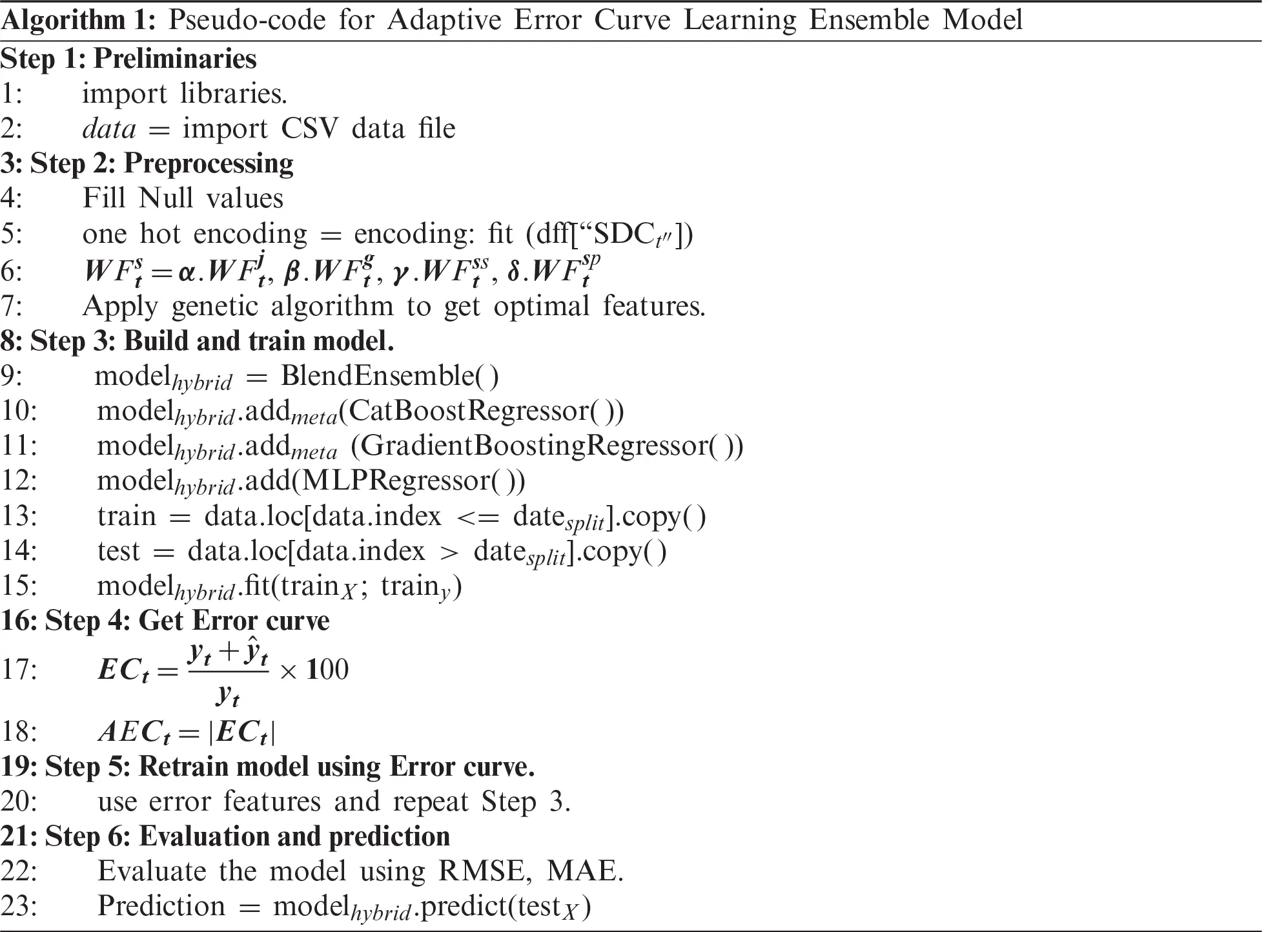4.1 Exploratory Data Analysis

This section provides the exploratory data analysis to understand the patterns of data better. The data provided by the Jeju energy corporation consist of different features. These features include the energy consumption of different energy sources, weather information of four weather stations, and holiday information. The total dataset consists of hourly-based energy consumption from 2012 to mid of 2019. Fig. 5 shows the monthly energy consumption in the complete dataset.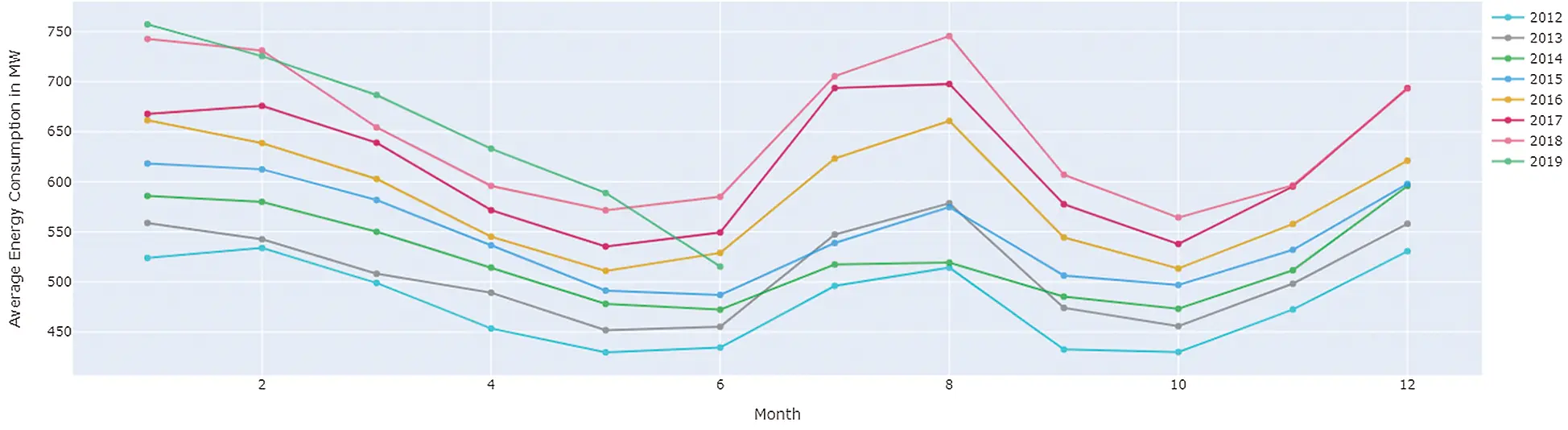Figure 5: Monthly average load consumption for each year

Each line shows a different year from 2012 to 2019. The X-axis represents the month, and Y-axis represents the average energy consumption in MWs. Average energy consumption is low in the month of November and high during August and January.

Fig. 6 shows the average weather temperate recorded at different Jeju island weather stations after applying weight factors. Each line shows a different year weather station’s data. 2019. The X-axis represents the time frame, and Y-axis represents the average temperature in Celsius.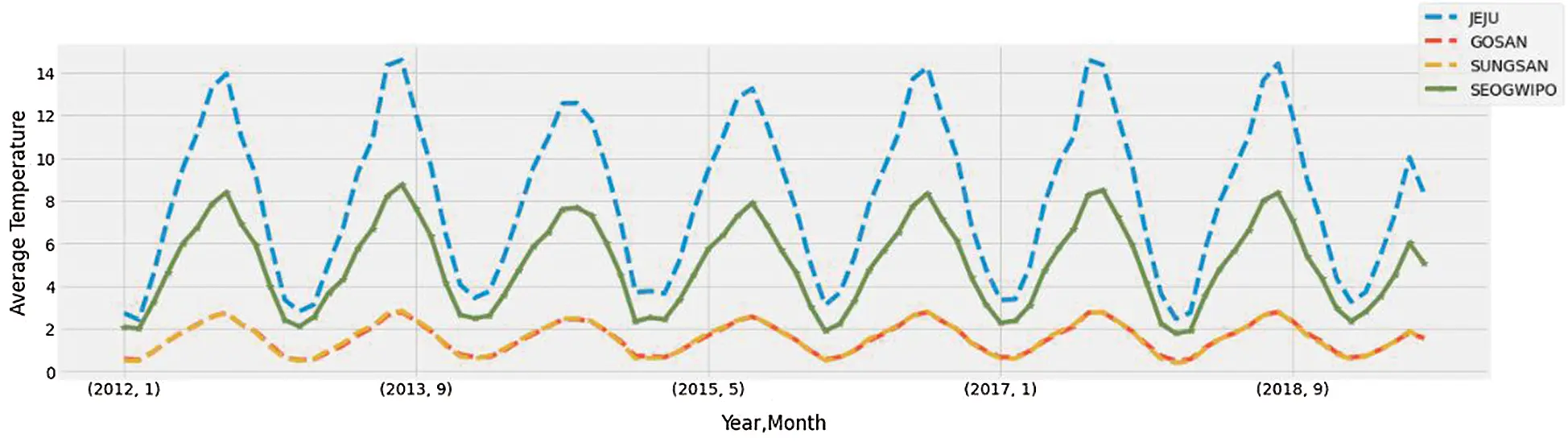Figure 6: Average temperature after applying weight for each weather station

Fig. 7 shows the energy consumption with respect to different seasons. Mean energy consumption is highest during the winter season and lowest during the spring season.Figure 7: Season wise average load consumption

Fig. 8 shows the mean energy consumption during each year. The gradual increase in consumption can be observed from this chart.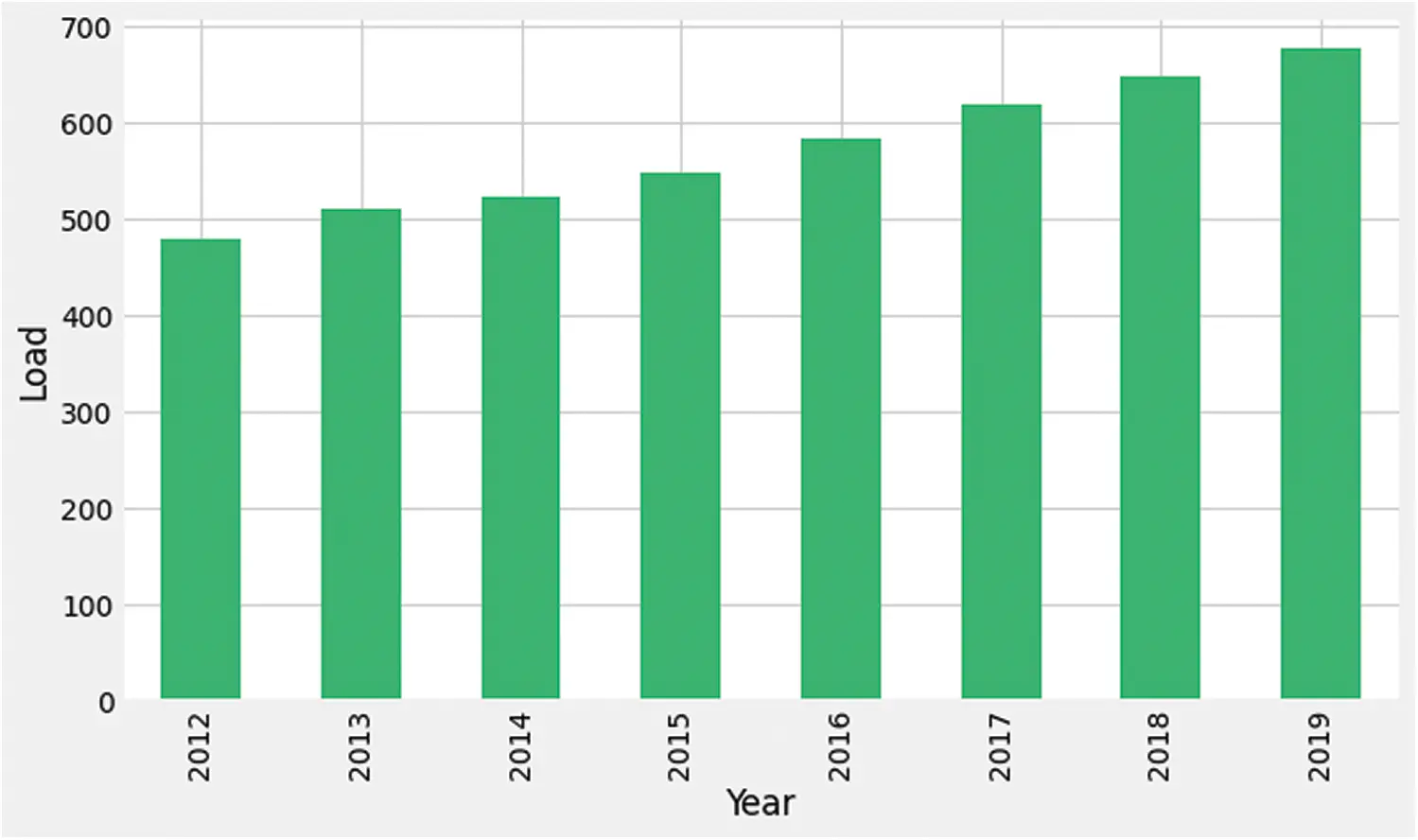Figure 8: Year-wise average load consumption

Tab. 1 summarizes the variable used in the dataset. It contains the variable name, their description, and measuring unit. Day of the week is represented from 0 to 6 starting from Sunday as 0. This table shows the input data consist of Code of Day of the Week, Holiday Code, Special Day Code, Special Day Name, Total Load, Temperature, Temperature of Due Point, Humidity, Wind Speed, Wind Direction Degree, Atmospheric Pressure on the Ground, Discomfort Index, Sensible Temperature, and Solar Irradiation Quantity. This data represents the problem adequately because it contains various weather and day features that have a great impact on the energy consumption.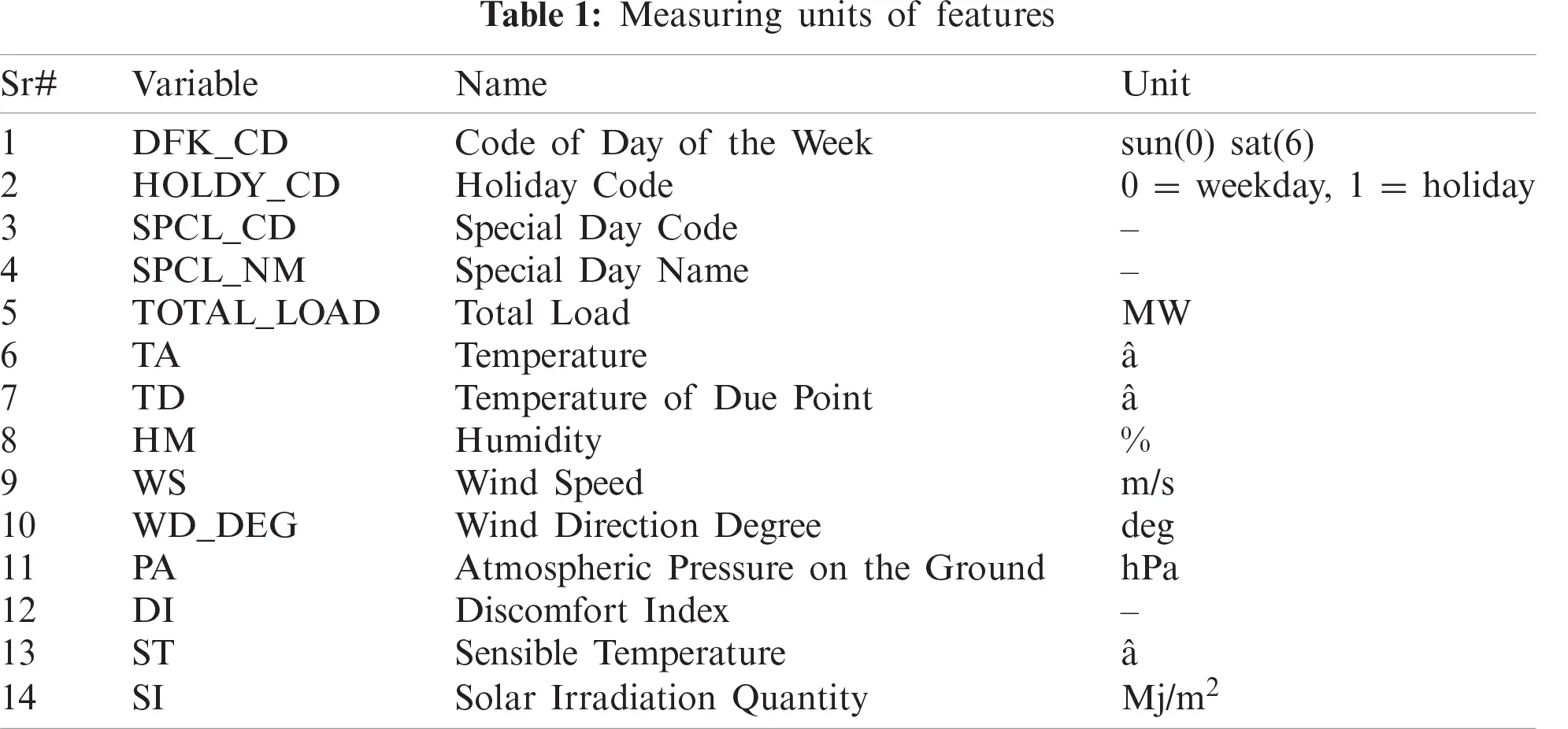Weekdays and holidays have assigned binary numbers, where weekday is represented as 0 and holiday as 1. Total load represents the hourly energy consumption in MW. Temperature, due point temperature, and moderate temperature are measured in Celsius. Other features used are humidity in percentage, wind speed in m/s, wind direction degree in deg, atmospheric pressure on the ground in hpa, and solar irradiation quantity in Mj/m2.

4.2 Training and Testing

The total dataset consists of hourly-based energy consumption from 2012 to 2019. It contains 64999 Rows. Tab. 2 summarizes the training and testing data for each phase.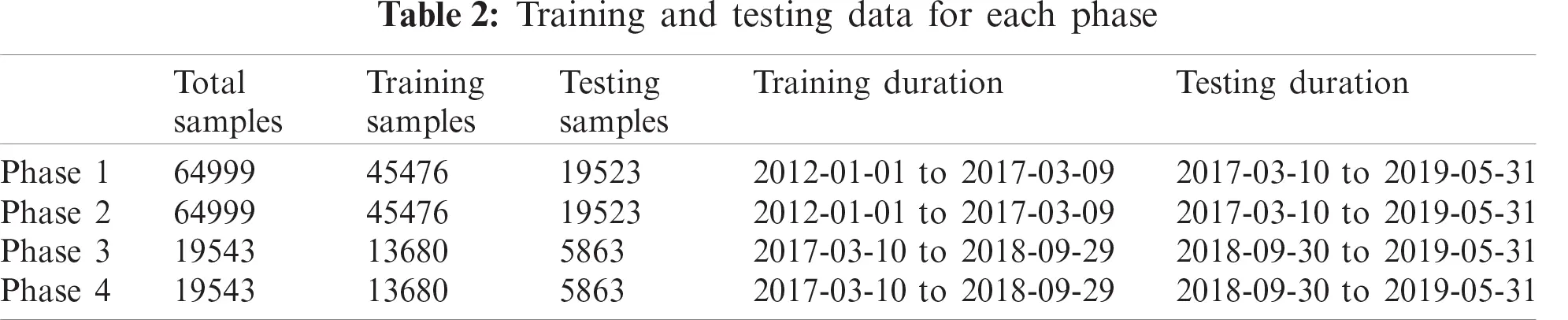Fig. 9 shows the graphical representation of testing and training data split for phase 1 and phase 2. The green color is used to represent the training data, and the blue color shows the testing phase data. For phase 1 and phase 2, we have used 62 months as training duration, which contains 45476 rows. The last 26 months are used as testing data and to obtain the error curve, which includes 19523 rows.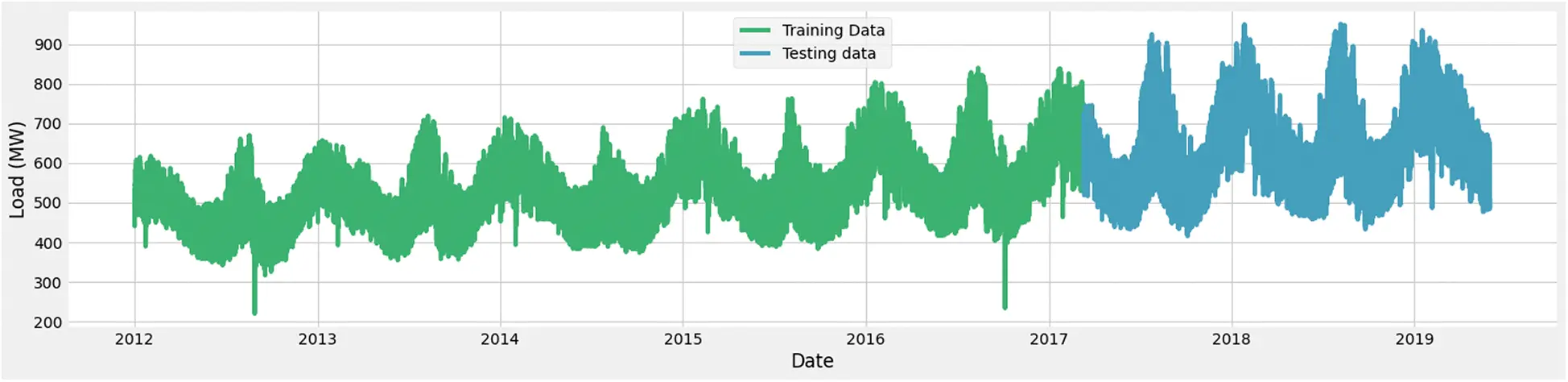Figure 9: Training and testing data for Phase 1 and Phase 2

The graphical representation of testing and training data split for phase 3 and phase 4 is shown in Fig. 10. For phase 3 and phase 4, we have used 18 months 20 days of the training data, consisting of 13680 rows from 10th of March 2017 to 29th of September 2018. We have used the remaining last months as testing data, consisting of 5763 rows from the 30th of September 2018 to the 31st of May 2019.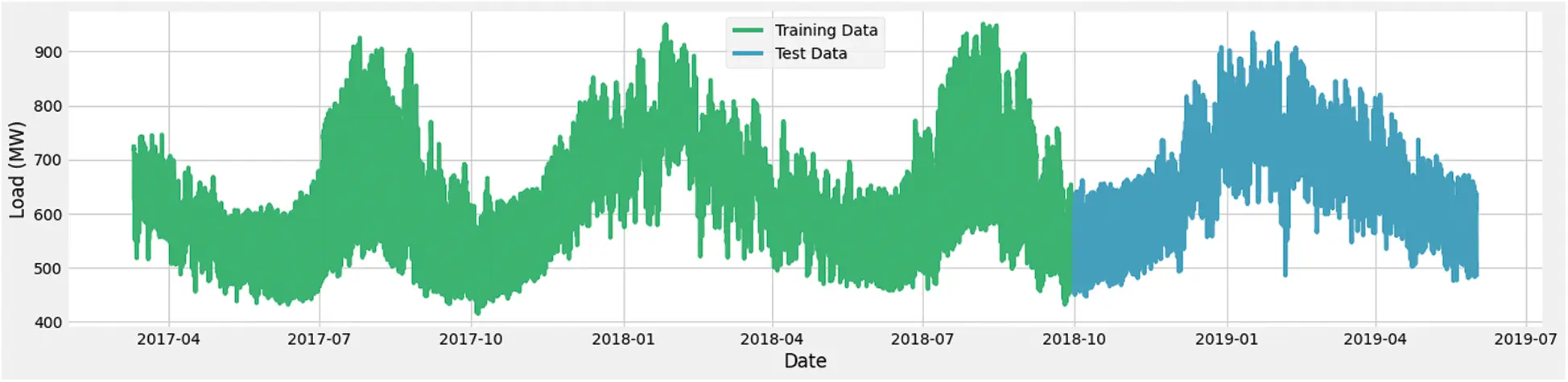Figure 10: Training and testing data for Phase 3 and Phase 4

5  Experimental Results and Evaluation

The accuracy of machine learning techniques must be verified before implementation in the real-world scenario . In this study, two methods are used to perform the accuracy and reliability of assessment procedures. One is a graphic visualization, and the other is error metrics. Error rating metrics used in the model evaluation method include mean absolute error (MAE), mean absolute percentage error (MAPE), root mean square error (RMSE), and root mean squared logarithmic error (RMSLE).

Fig. 11 shows the visual representation of Feature importance. The graph shows the order of features with respect to their importance after applying the genetic algorithm. The X-axis represents the feature importance score obtained using GA, and the Y-axis represents the feature’s name. The data is collected on an hourly basis, so it can be observed that the hour feature is of great importance in prediction, followed by the year feature. Then there are some weather features. We have removed the features with less importance to improve the results. There were 64 features, but after applying the genetic algorithm, the top 32 features are selected.

Fig. 12 shows the error curve obtained during phase 2. This error curve is used as an input feature along with other weather and date features during phase 4. This graph shows the variation in error on an hourly basis. The X-axis shows the month, and Y-axis shows the percentage of error.

MAE=1N (|ya-yp|) (14)

MSE=1N (ya-yp)2 (15)

RMSE=1N (ya-yp)2 (16)

RMSLE=1N (log(ya+1)-log(yp+1))2 (17)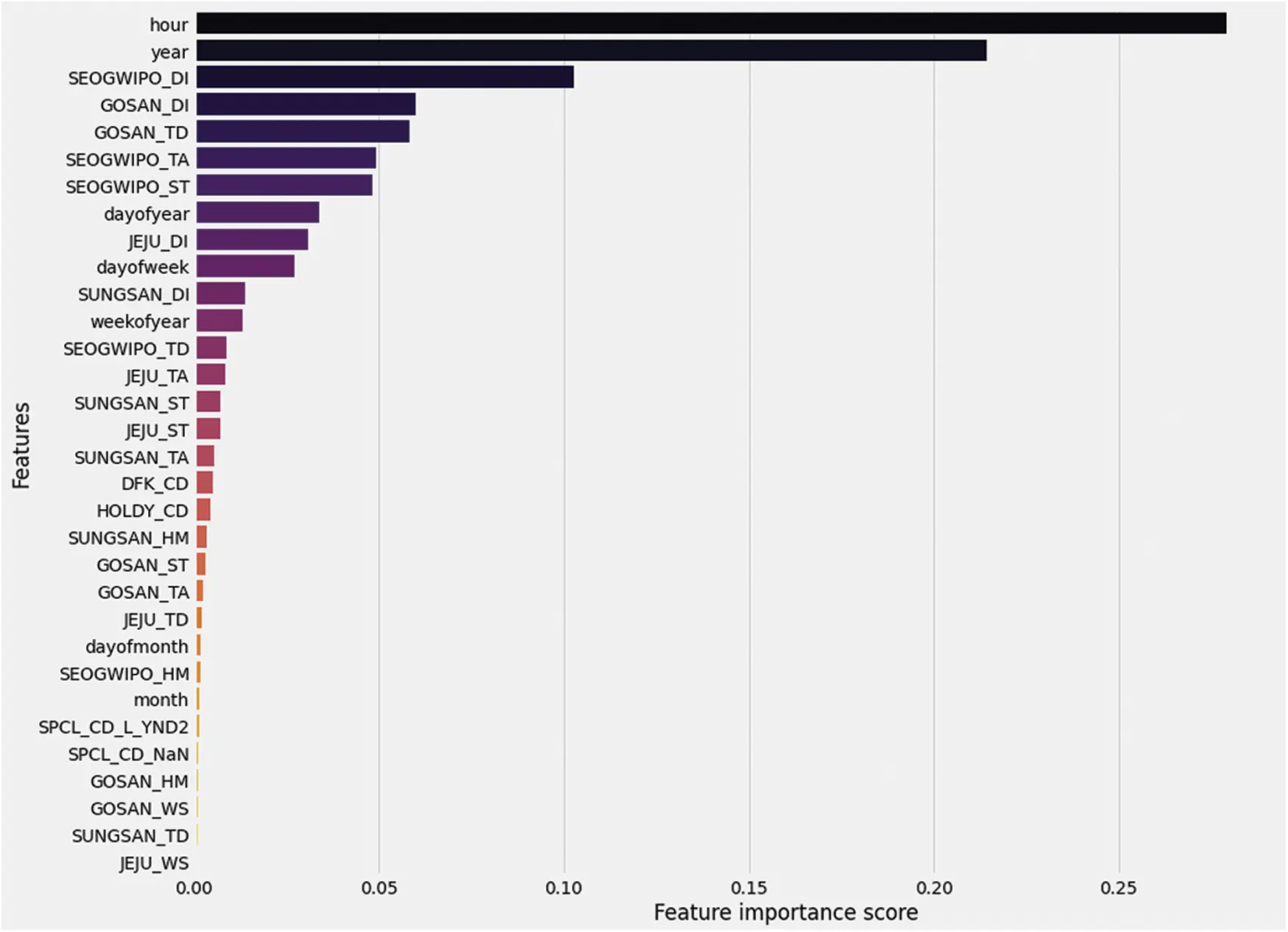Figure 11: Feature importance graph after applying genetic algorithm

The difference between prediction and actual values can be expressed as mean absolute error. It can be calculated using Eq. (14). Where yarepresents actual value and yp is the predicted value. First, we calculate all absolute errors, add all up, and then divide by the total number of errors expressed in the Eq. as N. The proposed model’s MAE is 3.05. Mean squared error is calculated using Eq. (15). It represents the difference between the original value and the expected value . First, we calculate all absolute errors, take the square of them, and then divide by the total number of errors. The MSE of the proposed model is 115.09. RMSE is obtained by Eq. (16), It is calculated by the root of MSE. Where yp is the forecasted value obtained by the proposed model, and ya is the actual load value. N represents the sample size in terms of numbers . The RMSE of the proposed model is 5.05.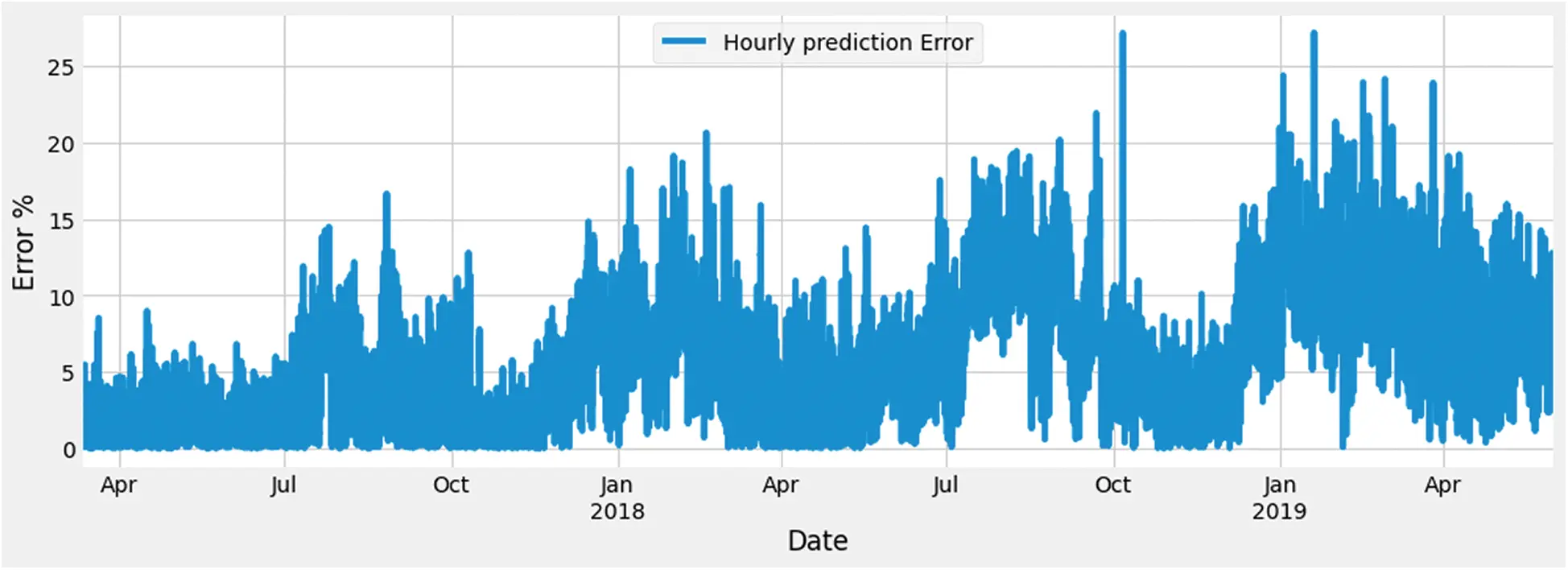Figure 12: Error curve generated from phase 2

The root mean squared logarithmic error (RMSLE) is obtained by Eq. (17). The root mean squared logarithmic error expressed the logarithmic relationship between the actual data value and the model’s expected value. The RMSLE of the proposed model is 0.008.

Tab. 3 summarizes the error during all phases of experimental results. It covers the minimum, maximum, and mean error of testing data according to each phase. From the table, it is evident that there is a significant improvement in the results when we applied ECL. We have obtained a maximum of 10.80% error, and the mean error recorded was 1.22%. We also performed a comparison of the proposed GA-ECLE model with the state-of-the-art models including. Tab. 4 shows the comparison of different evaluation metrics.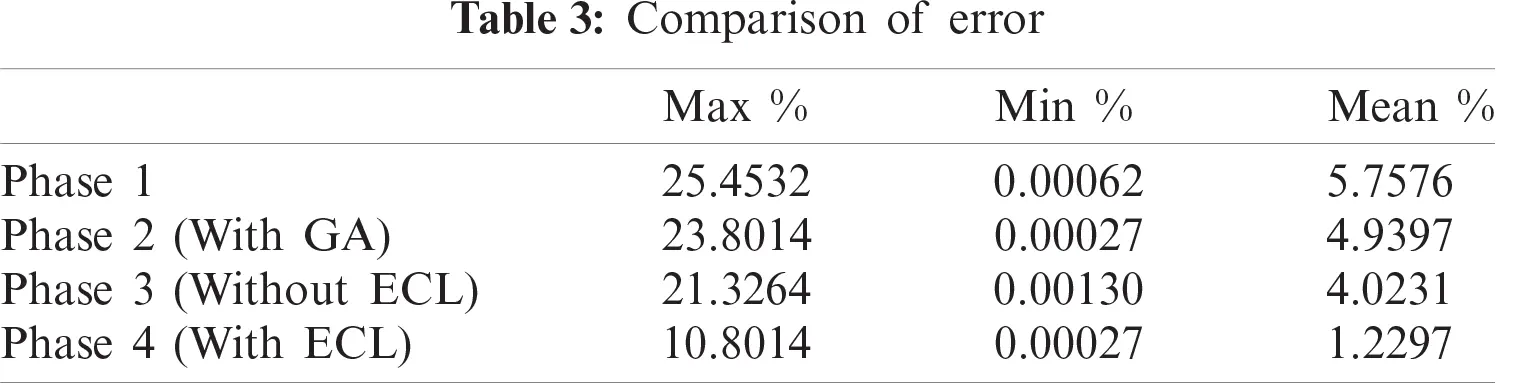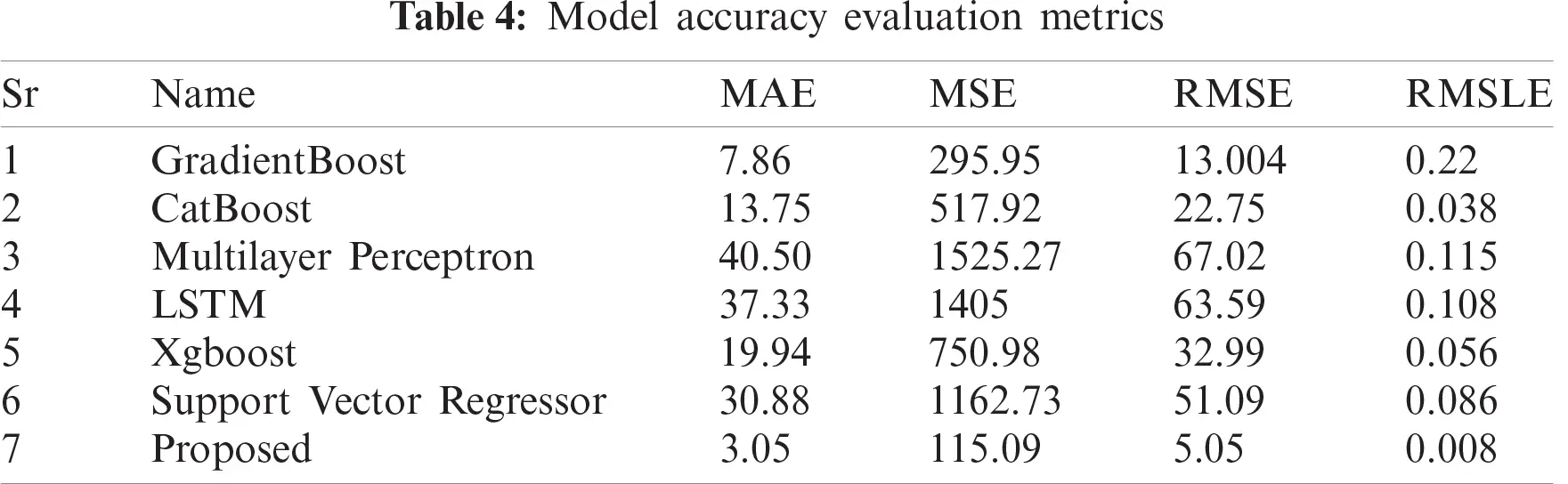The prediction result of testing data is also illustrated using graphs. In Phase 4, data from the 30th of September 2018 to the 31st of May 2019 is used as testing data. Fig. 13 represents the graphical comparison between the results of applying ECL.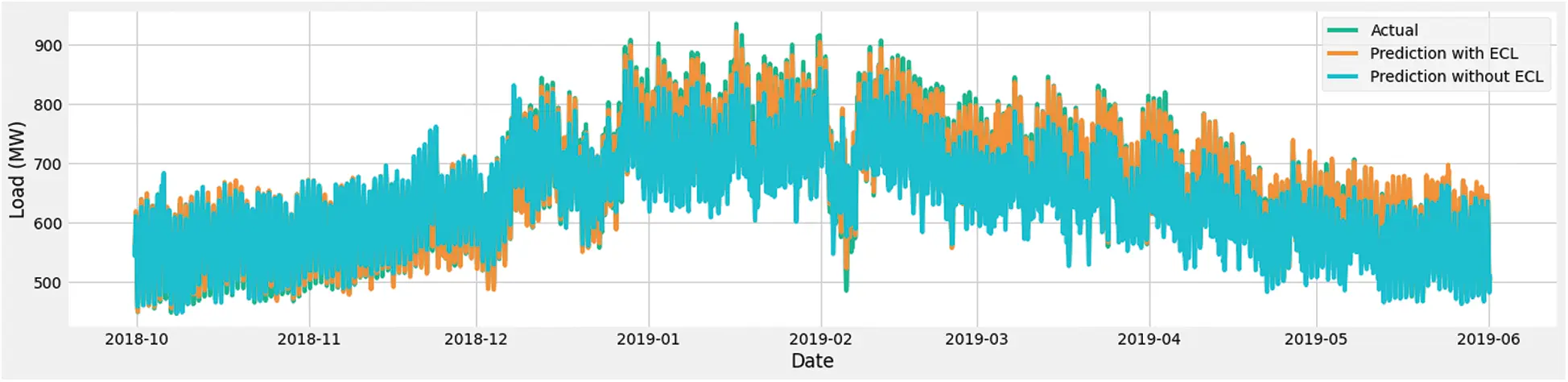Figure 13: Comparison of Actual test data and prediction with and without ECL

Green lines show the actual load values, orange lines show the prediction result after applying ECL, and cyan color lines represent the prediction using the same model but without ECL.

To better visualize the results, we have selected two different time frames. One is 48 h, and the other is one week or 168 h. Fig. 14 shows the results between 48 h, which comprised from the 20th of May 2019 to the 22nd of May 2019. Fig. 15 shows the graphical representation of actual predictions and their difference for one week. The week we selected starts on the 15th of February 2019 and ends on the 21st of February 2019. In both figures, the green line shows the actual load consumption, and the orange line represents the prediction with ECL. Whereas the difference in prediction and actual consumption is illustrated using purple color.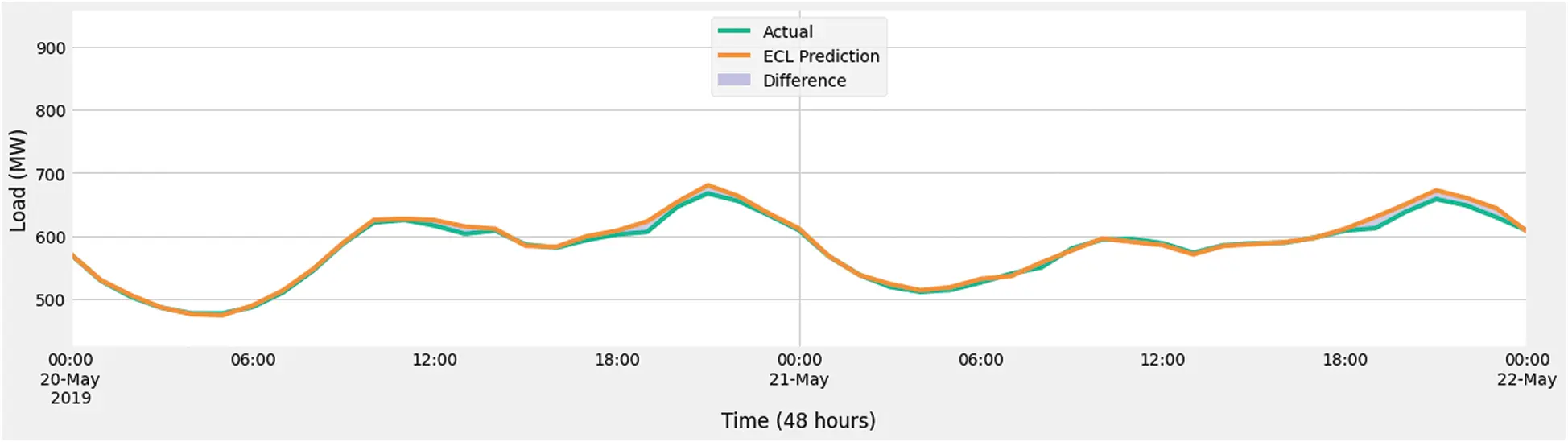Figure 14: Comparison of 48 h ahead prediction with and without ECLFigure 15: Comparison of the week ahead prediction with and without ECL

6  Conclusions

This research presents a novel genetic algorithm-based adaptive error curve learning ensemble model. The proposed technique sorts random variants to improve energy consumption predictions according to a machine learning-based ensembled approach. The modified ensemble model use error as a function to improve prediction accuracy. This approach combines three models: CatBoost, Gradient Boost, and Multilayer Perceptron. A genetic algorithm is used to obtain the best properties available in the model. To prove the proposed model’s effectiveness, we used a four-step technique that utilized Jeju Island’s actual energy consumption data. In the first step, the CB-GB-MLP model was applied, and the results were obtained. In the second phase, we used a large, full-featured GA model. The third step is to compare the energy prediction results with the proposed ECL model. The fourth step is the final step of applying the GA-ECLE model. Extensive experimental results have been presented to show that the proposed GA-ECLE model is superior to the existing machine learning models such as GradientBoost, CatBoost, Multilayer Perceptron, LSTM, Xgboost, and Support Vector Regressor. The results of our approach seem very promising. We obtained a mean error of 1.22%, which was 5.75%, without using the proposed approach. We have presented the results in a graphical way along with the statistical comparison. The empirical results seem mostly favorable for the applicability of the proposed model in the industry. In the future, other features can be added, such as the impact of the population using electricity and the number of electric vehicles.

Funding Statement: This research was financially supported by the Ministry of Small and Medium-sized Enterprises (SMEs) and Startups (MSS), Korea, under the “Regional Specialized Industry Development Program (R&D, S2855401)” supervised by the Korea Institute for Advancement of Technology (KIAT).

Conflicts of Interest: The authors declare that they have no conflicts of interest to report regarding the present study.

## References

1. G. Alova, P. A. Trotter and A. Money, “A machine-learning approach to predicting Africa’s electricity mix based on planned power plants and their chances of success,” Nature Energy, vol. 6, no. 2, pp. 158–166, 202
2. X. Shao, C. S. Kimand and D. G. Kim, “Accurate multi-scale feature fusion cnn for time series classification in smart factory,” Computers, Materials & Continua, vol. 65, no. 1, pp. 543–561, 2020.
3. D. Zhou, S. Ma, J. Hao, D. Han, D. Huang et al., “An electricity load forecasting model for integrated energy system based on bigan and transfer learning,” Energy Reports, vol. 6, pp. 3446–3461, 2020.
4. H.-Y. Su, T.-Y. Liu and H.-H. Hong, “Adaptive residual compensation ensemble models for improving solar energy generation forecasting,” IEEE Transactions on Sustainable Energy, vol. 11, no. 2, pp. 1103–1105, 2019.
5. C. Schön, J. Dittrich and R. Müller, “The error is the feature: How to forecast lightning using a model prediction error,” in Proc. of the 25th ACM SIGKDD Int. Conf. on Knowledge Discovery & Data Mining, 2019.
6. J. Zhang, W. Xiao, Y. Li and S. Zhang, “Residual compensation extreme learning machine for regression,” Neurocomputing, vol. 311, pp. 126–136, 2018.
7. J.-H. Won, Lee, J.-W. Kim and G.-W. Koh, “Groundwater occurrence on Jeju island, Korea,” Hydrogeology Journal, vol. 14, no. 4, pp. 532–547, 2006.
8. C. Li, “Designing a short-term load forecasting model in the urban smart grid system,” Applied Energy, vol. 266, pp. 114850, 2020.
9. F. Zhang, B. Du and L. Zhang, “Scene classification via a gradient boosting random convolutional network framework,” IEEE Transactions on Geoscience and Remote Sensing, vol. 54, no. 3, pp. 1793–1802, 2015.
10. J. Lu, D. Lu, X. Zhang, Y. Bi, K. Cheng et al., “Estimation of elimination half-lives of organic chemicals in humans using gradient boosting machine,” Biochimica et Biophysica Acta (BBA)-General Subjects, vol. 1860, no. 11, pp. 2664–2671, 2016.
11. X. Lei and Z. Fang, “Gbdtcda: Predicting circrna-disease associations based on gradient boosting decision tree with multiple biological data fusion,” International Journal of Biological Sciences, vol. 15, no. 13, pp. 2911, 2019.
12. H. Lu, F. Cheng, X. Ma and G. Hu, “Short-term prediction of building energy consumption employing an improved extreme gradient boosting model: A case study of an intake tower,” Energy, vol. 203, pp. 117756, 2020.
13. Y. Zhang and A. Haghani, “A gradient boosting method to improve travel time prediction,” Transportation Research Part C: Emerging Technologies, vol. 58, pp. 308–324, 2015.
14. S. Touzani, J. Granderson and S. Fernandes, “Gradient boosting machine for modeling the energy consumption of commercial buildings,” Energy and Buildings, vol. 158, pp. 1533–1543, 2018.
15. P. W. Khan, Y.-C. Byun, S.-J. Lee, D.-H. Kang, J.-Y. Kang et al., “Machine learning-based approach to predict energy consumption of renewable and non-renewable power sources,” Energies, vol. 13, no. 18, pp. 4870, 2020.
16. A. V. Dorogush, V. Ershov and A. Gulin, “Catboost: Gradient boosting with categorical features support,” arXiv preprint arXiv: 1810.11363, 2018.
17. L. Diao, D. Niu, Z. Zang and C. Chen, “Short-term weather forecast based on wavelet denoising and catboost,” in Chinese Control Conf., Guangzhou, China, IEEE, 2019.
18. P. Wang, B. A. Hafshejani and D. Wang, “An improved multi-layer perceptron approach for de-tecting sugarcane yield production in iot based smart agriculture,” Microprocessors and Microsystems, vol. 82, pp. 103822, 2021.
19. S. Saha, G. C. Paul, B. Pradhan, K. N. Abdul Maulud, A. M. Alamri et al., “Integrating multi-layer perceptron neural nets with hybrid ensemble classifiers for deforestation probability assessment in eastern India,” Geomatics Natural Hazards and Risk, vol. 12, no. 1, pp. 29–62, 2021.
20. A. A. Tamouridou, T. K. Alexandridis, X. E. Pantazi, A. L. Lagopodi, J. Kashefi et al., “Application of multi-layer perceptron with automatic relevance determination on weed mapping using UAV multispectral imagery,” Sensors, vol. 17, no. 10, pp. 2307, 2017.
21. P. W. Khan and Y.-C. Byun, “Genetic algorithm based optimized feature engineering and hybrid machine learning for effective energy consumption prediction,” IEEE Access, vol. 8, pp. 196274–196286, 2020.
22. M. K. Amjad, S. I. Butt, R. Kousar, R. Ahmad, M. H. Agha et al., “Recent research trends in genetic algorithm based flexible job shop scheduling problems,” Mathematical Problems in Engineering, vol. 2018, 2018.
23. X. Wu, Y. Wang, Y. Bai, Z. Zhu, A. Xia et al., “Online short-term load forecasting methods using hybrids of single multiplicative neuron model, particle swarm optimization variants and nonlinear filters,” Energy Reports, vol. 7, pp. 683–692, 2021.
24. S. Goda, N. Agata and Y. Matsumura, “A stacking ensemble model for prediction of multi-type tweet engagements,” in Proc. of the Recommender Systems Challenge 2020, Brazil, pp. 6–10, 2020.
25. N. Somu, G. R. MR and K. Ramamritham, “A hybrid model for building energy consumption forecasting using long short term memory networks,” Applied Energy, vol. 261, pp. 114131, 2020.
26. M. Massaoudi, S. S. Refaat, I. Chihi, M. Trabelsi, F. S. Oueslati et al., “A novel stacked generalization ensemble-based hybrid lgbm-xgb-mlp model for short-term load forecasting,” Energy, vol. 214, pp. 118874, 2020.
27. K. Park, S. Yoon and E. Hwang, “Hybrid load forecasting for mixed-use complex based on the characteristic load decomposition by pilot signals,” IEEE Access, vol. 7, pp. 12297–12306, 2019.
28. P. W. Khan, Y.-C. Byun, S.-J. Lee and N. Park, “Machine learning based hybrid system for imputation and efficient energy demand forecasting,” Energies, vol. 13, no. 11, pp. 2681, 2020.
29. G. A. Tahir and C. K. Loo, “An open-ended continual learning for food recognition using class incremental extreme learning machines,” IEEE Access, vol. 8, pp. 82328–82346, 2020.
30. Z. Wang, T. Zhang, Y. Shao and B. Ding, “LSTM-Convolutional-BLSTM encoder-decoder network for minimum mean-square error approach to speech enhancement,” Applied Acoustics, vol. 172, pp. 107647, 2021.
31. R. Ünlü and E. Namlı, “Machine learning and classical forecasting methods-based decision support systems for covid-19,” Computers, Materials & Continua, vol. 64, no. 3, pp. 1383–1399, 2020.This work is licensed under a Creative Commons Attribution 4.0 International License, which permits unrestricted use, distribution, and reproduction in any medium, provided the original work is properly cited.